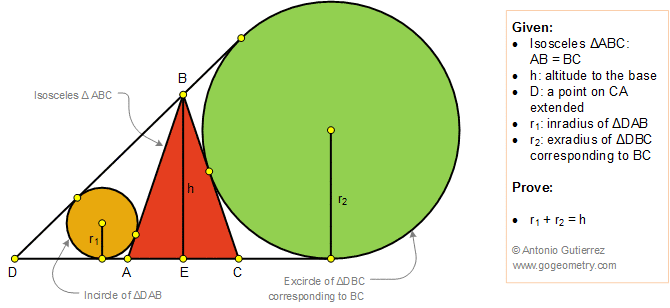# Geometry Problem 1373: Isosceles Triangle, Exterior Cevian, Inradius, Exradius, Altitude to the Base.

### Proposition

In the figure below, ABC is an isosceles triangle (AB = BC) with altitude BE = h. D is a point on CA extended, r1 is the inradius of the triangle DAB and r2 is the exradius of the triangle DBC corresponding to BC. Prove that r1 + r2 = h.

BD is called an exterior cevian of triangle ABC.